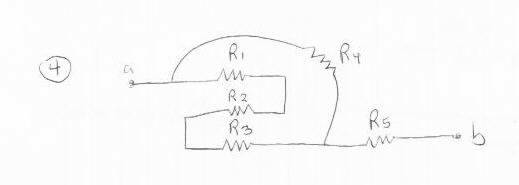# Circuit analysis - Potential differences of resistors

Baou

## Homework Statement$$R_1 = 70 \Omega$$, $$R_2 = 144 \Omega$$, $$R_3 = 237 \Omega$$, $$R_4 = 117 \Omega$$, $$R_5 = 246 \Omega$$; $$V_{ab}=43 V$$
I am looking for the voltage of each resistor as well as the current passing through each one.

## Homework Equations

Kirchoff's Voltage/Current Laws: The sum of any potential differences across a closed path is zero; current (charge) is conserved, so any current exiting a node is equal to the sum of the currents entering it.
$$V = IR$$
$$I = \frac VR$$

## The Attempt at a Solution

I know $$R_{eq}=339\Omega$$. Thus, $$I = \frac VR = \frac{43}{339} = .127 A$$.

$$V_5 = I*R_5=.127*246=31.2 V$$

$$V_1+V_2+V_3-V_4=0$$. This is Kirchoff's voltage equation.

$$I_1=I_2=I_3$$ b/c current is conserved, so $$\frac{V_1}{70}=\frac{V_2}{144}=\frac{V_3}{237}$$. I solve for $$V_1$$ and $$V_2$$ in terms of $$V_3$$: $$V_1=\frac{70V_3}{237}$$ and $$V_2=\frac{144V_3}{237}$$.

I know the current through $$V_3$$ and $$V_4$$ should sum to .127, so: $$\frac{V_3}{237}+\frac{V_4}{117}=.127$$.
$$V_4=117\left(.127-\frac{V_3}{237}\right)$$.

$$V_4=V_1+V_2+V_3$$ from above, so plugging in everything I get:
$$117\left(.127-\frac{V_3}{237}\right)=\frac{70V_3}{237}+\frac{144V_3}{237}+V_3$$.

I solve for $$V_3$$ and then plug that in to everything else.
My final answers are: $$V_1 = 1.83$$, $$V_2=3.77$$, $$V_3=6.2$$, $$V_4=12.8$$, $$V_5=31.2$$. However, these answers are incorrect, so I was hoping to find out what I'm doing wrong.